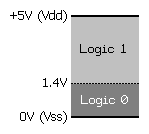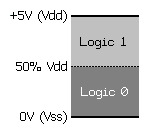TTL   (SX48/52 Only)

TTL Pin {, Enable}

Function
Configures Pin for TTL input threshold (1.4 volts) on the SX48 or SX52. This command does not apply to the SX18, SX20, or SX28 (use the LVL_A, LVL_B, and LVL_C registers).

• Pin  is any SX48/52 I/O pin (RA.0 .. RA.7, RB.0 .. RB.7, RC.0 .. RC.7, RD.0 .. RD.7, RE.0 .. RE.7).
• Enable is a constant, 0 or 1, that enables (1) or disables (0) the TTL input threshold. When not specified, Enable defaults to 1. If Enable is 0, the pin will be configured for CMOS input threshold.

Explanation
Every I/O pin has selectable logic level control that determines the voltage threshold for a logic level 0 or 1. The default logic level for all I/O pins is TTL but can be modified by writing to the appropriate logic-level register (LVL_A, LVL_B, LVL_C, LVL_D and LVL_E). The logic level can be configured for all pins, regardless of pin direction, but really matters only when the associated pin is set to input mode. By configuring logic levels on input pins, the SX chip can be sensitive to both TTL and CMOS logic thresholds. The figure below demonstrates the difference between TTL and CMOS logic levels.TTL Logic Level CMOS Logic Level

The logic threshold for TTL is 1.4 volts; a voltage below 1.4 is considered to be a logic 0, while a voltage above is considered to be a logic 1. The logic threshold for CMOS is 50% of Vdd, a voltage below Ẅ Vdd is considered to be a logic 0, while a voltage above Ẅ Vdd is considered to be a logic 1.

```Start:
TTL RE.7, 1                                   ' set to TTL level
TTL RE.6                                      ' set to TTL level
TTL RE.5, 0                                   ' disable TTL level, set to CMOS level
```

Related instructions: CMOS, PULLUP, and SCHMITT